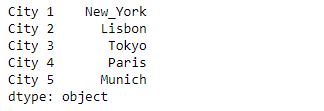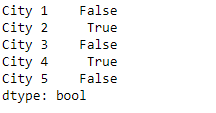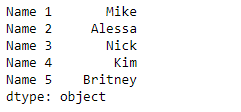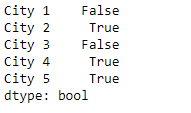Open In App

# Python | Pandas Series.str.contains()

Series.str can be used to access the values of the series as strings and apply several methods to it. Pandas Series.str.contains() function is used to test if pattern or regex is contained within a string of a Series or Index. The function returns boolean Series or Index based on whether a given pattern or regex is contained within a string of a Series or Index.

Syntax: Series.str.contains(pat, case=True, flags=0, na=nan, regex=True)
Parameter :
pat : Character sequence or regular expression.
case : If True, case sensitive.
flags : Flags to pass through to the re module, e.g. re.IGNORECASE.
na : Fill value for missing values.
regex : If True, assumes the pat is a regular expression.
Returns : Series or Index of boolean values

Example #1: Use Series.str.contains a () function to find if a pattern is present in the strings of the underlying data in the given series object.

## Python3

 `# importing pandas as pd``import` `pandas as pd` `# importing re for regular expressions``import` `re` `# Creating the Series``sr ``=` `pd.Series([``'New_York'``, ``'Lisbon'``, ``'Tokyo'``, ``'Paris'``, ``'Munich'``])` `# Creating the index``idx ``=` `[``'City 1'``, ``'City 2'``, ``'City 3'``, ``'City 4'``, ``'City 5'``]` `# set the index``sr.index ``=` `idx` `# Print the series``print``(sr)`

Output :Now we will use Series.str.contains a () function to find if a pattern is contained in the string present in the underlying data of the given series object.

## Python3

 `# find if 'is' substring is present``result ``=` `sr.``str``.contains(pat ``=` `'is'``)` `# print the result``print``(result)`

Output :As we can see in the output, the Series.str.contains() function has returned a series object of boolean values. It is true if the passed pattern is present in the string else False is returned.
Example #2: Use Series.str.contains a () function to find if a pattern is present in the strings of the underlying data in the given series object. Use regular expressions to find patterns in the strings.

## Python3

 `# importing pandas as pd``import` `pandas as pd` `# importing re for regular expressions``import` `re` `# Creating the Series``sr ``=` `pd.Series([``'Mike'``, ``'Alessa'``, ``'Nick'``, ``'Kim'``, ``'Britney'``])` `# Creating the index``idx ``=` `[``'Name 1'``, ``'Name 2'``, ``'Name 3'``, ``'Name 4'``, ``'Name 5'``]` `# set the index``sr.index ``=` `idx` `# Print the series``print``(sr)`

Output :Now we will use Series.str.contains a () function to find if a pattern is contained in the string present in the underlying data of the given series object.

## Python3

 `# find if there is a substring such that it has``# the letter 'i' followed by any small alphabet.``result ``=` `sr.``str``.contains(pat ``=` `'i[a-z]'``, regex ``=` `True``)` `# print the result``print``(result)`

Output :As we can see in the output, the Series.str.contains() function has returned a series object of boolean values. It is true if the passed pattern is present in the string else False is returned.